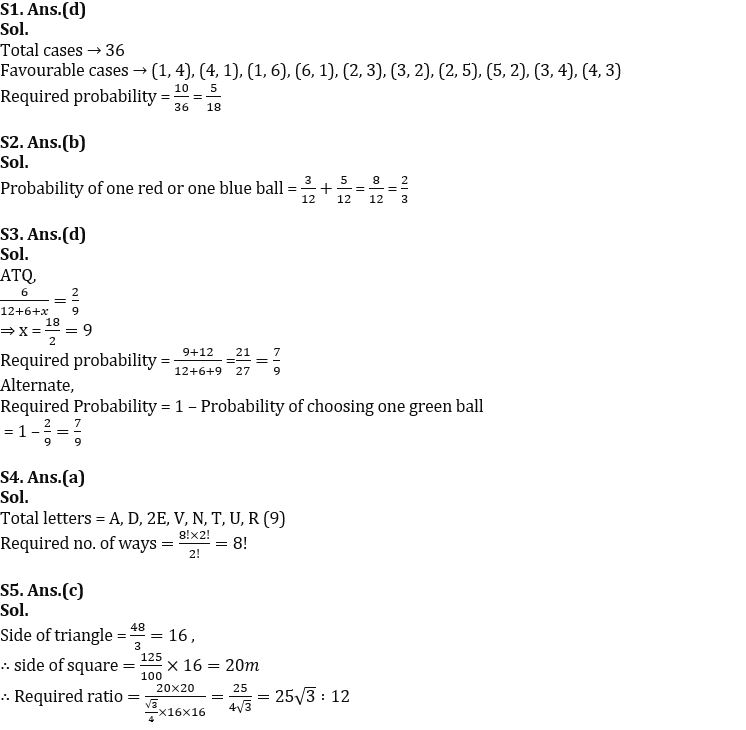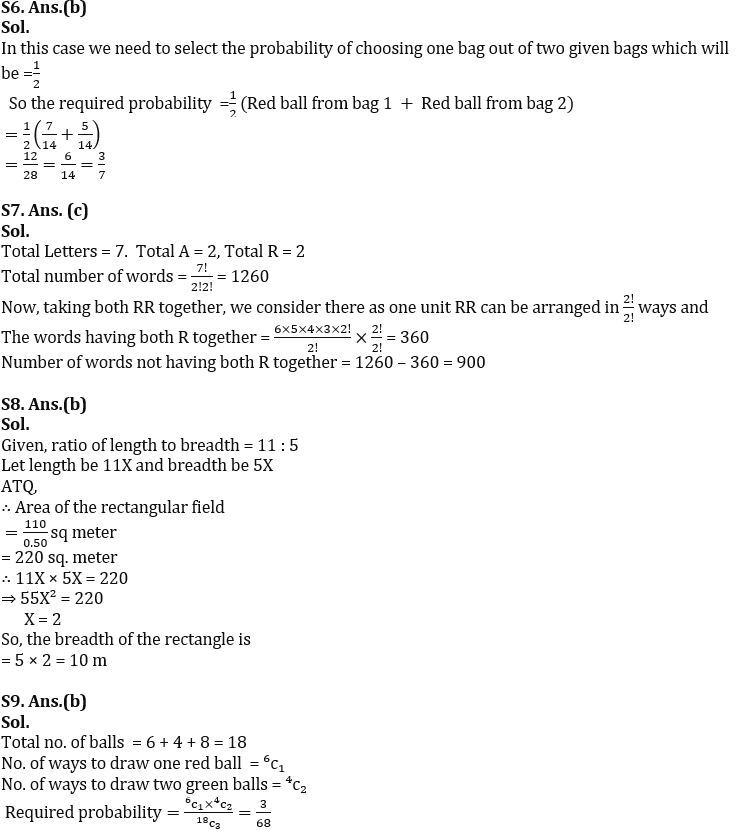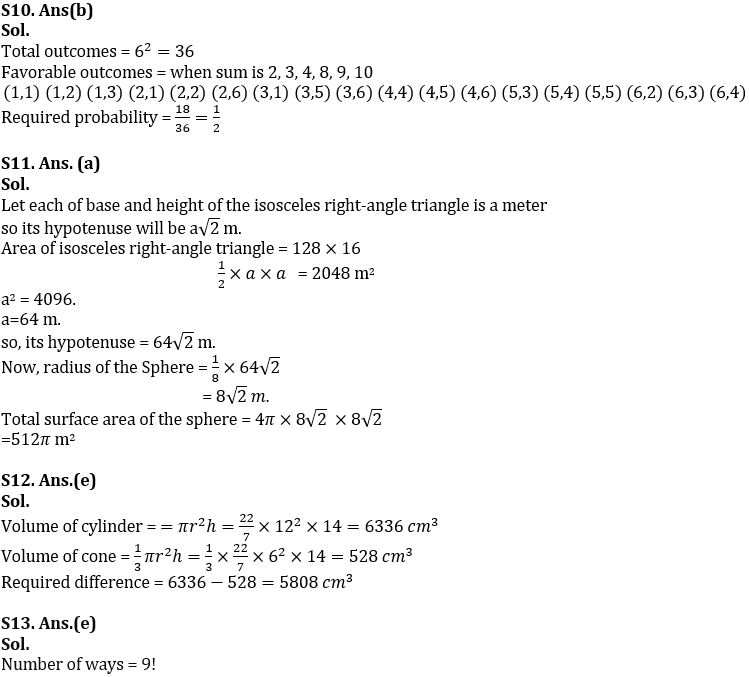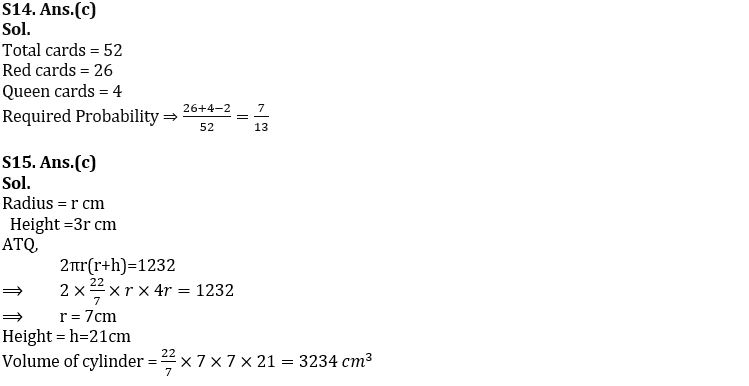Latest Banking jobs   »

# Quantitative Aptitude Quiz For IBPS RRB PO/Clerk Prelims 2022- 29th July

Q1. A man have two dice, if he rolled both dice, then find the probability that sum of digits will be 5 or 7 ?
(a) 1/18
(b) 1/12
(c) 5/9
(d) 5/18
(e) 7/18

Q2. A bag contains 3 red, 5 blue and 4 green ball. 1 ball is drawn at random. What is the probability of ball being either red or blue colour.
(a) 3/7
(b) 2/3
(c) 5/12
(d) 1/3
(e) 1/4

Q3. A box contains 12 red, 6 green and ‘x’ yellow balls. Probability of choosing one green ball out of the box is 2/9, then find the probability of choosing one ball which can be either red or yellow?
(a) 4/9
(b) 5/9
(c) 2/3
(d) 7/9
(e) 8/9

Q4. In how many different ways the letters of the word ‘ADVENTURE’ can be arranged such that ‘NT’ always come together?
(a) 8!
(b) 7!
(c) 9!
(d) 9! /2!
(e) 8! /2!

Q5. The side of a square is 25% more than the side of an equilateral triangle whose perimeter is 48 m. Find the ratio between area of square to area of triangle.
(a) 25 : 12
(b) 25√2 : 12
(c) 25√3 : 12
(d) 20√3 : 12
(e) 11√3 : 12

Q6. A bag has seven red, four white and three green balls while another bag has five red, six yellow and three blue balls. A bag is selected at random and a ball drawn out of it, then Find the probability that the ball drawn is red.
(a) 1/7
(b) 3/7
(c) 2/7
(d) 1
(e) 6/7

Q7. Find the number of ways in which the letters of the word ARRANGE can be arranged such that both R do not come together.
(a) 950
(b) 800
(c) 900
(d) 750
(e) 920

Q8. A rectangular field cost Rs. 110 for leveling at 50 paise per square meter. If the ratio of length to breadth is 11: 5. Find the breadth of field ?
(a) 12 m
(b) 10 m
(c) 5 m
(d) 16 m
(e) 15 m

Q9. A bag contains 6 red balls, 4 green balls and 8 white balls. If three balls are drawn at random, find the probability that one is red ball and two are green balls?
(a) 3/69
(b) 3/68
(c) 3/65
(d) 4/67
(e) 3/71

Q10. If two dice are rolled simultaneously, find the probability of obtaining the sum (of numbers on these two dices) which is divisible by 2 or 3 but not by both?
(a)1/4
(b) 1/2
(c) 1/5
(d) 1/6
(e) 1/3

Q11. The area of a rectangular field having length 128m and breadth 16m is equal to the area of an isosceles right-angle triangle. If the radius of a sphere is 12 ½ % of the hypotenuse of the isosceles right-angle triangle, then find out the total surface area of sphere?
(a) 512π m²
(b) 343π m²
(c) 580π m²
(d) 494π m²
(e) 500π m²

Q12. A cylindrical solid toy (radius 12cm) is melted and poured into a beaker to form a solid cone. Due to splashing some molten metal splash outside the beaker so the volume of the cone reduced. Find the volume of molten metal that splash outside of the beaker if height of both cylinder and cone is 14cm while radius of cone is half of that of cylinder.
(a) 1056 cm³
(b) 2244 cm³
(c) 3300 cm³
(d) 4356 cm³
(e) 5808 cm³

Q13. Find the no. of way to arrange the letters of word ‘ EDUCATION ’.
(a) 8!/2
(b) 10!/2
(c) 6!/2
(d) 7!/2
(e) 9!

Q14. What is the probability of finding a red card or a queen from a well shuffled pack of 52 cards.
(a) 15/26
(b) 7/12
(c) 7/13
(d) 5/13
(e) 8/13

Q15. The total surface area of a cylindrical vessel is 1232 cm² and the height of vessel is 2 times more than the radius of vessel. Find the volume of cylindrical vessel?
(a) 4312 cm³
(b) 3201 cm³
(c) 3234 cm³
(d) 3256 cm³
(e) 3333 cm³

Solutions#### Congratulations!Incorrect details? Fill the form again here

•Quantitative Aptitude Quiz For IBPS RRB ...
•Reasoning Ability Quiz For IBPS RRB PO C...
•Quantitative Aptitude Quiz For IBPS RRB ...
•Reasoning Ability Quiz For IBPS RRB PO C...
•Quantitative Aptitude Quiz For IBPS RRB ...
•Reasoning Ability Quiz For IBPS RRB PO C...